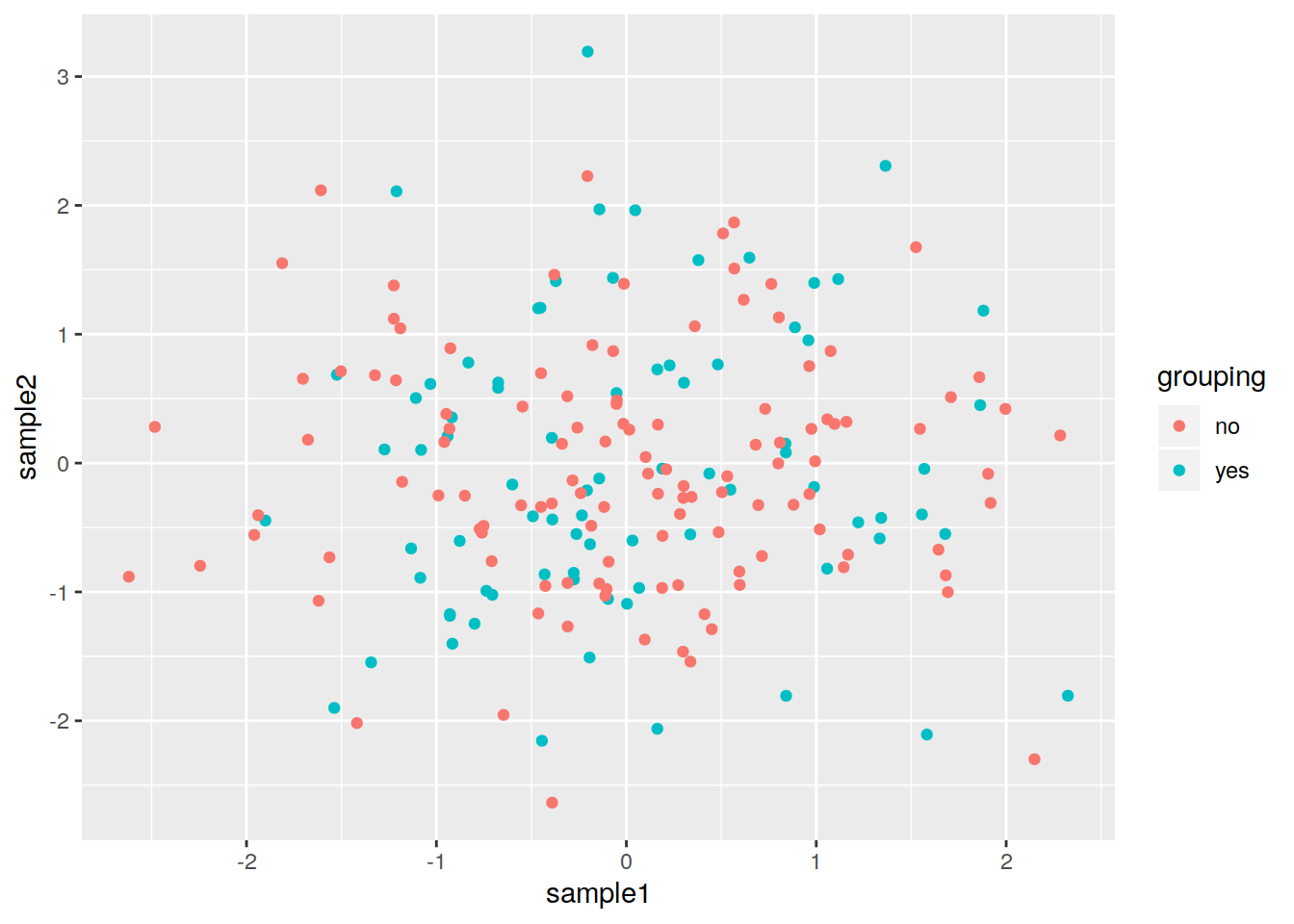## 19.2 Scatter plot

``````# Example of a scatter plot: add the geom_point() layer
ggplot(data=dataframe, mapping=aes(x=column1, y=column2)) + geom_point()``````
• Example of a simple scatter plot:
``````# Create a data frame
df1 <- data.frame(sample1=rnorm(200), sample2=rnorm(200))

# Plot !
ggplot(data= df1 , mapping=aes(x=sample1, y=sample2)) +
geom_point()``````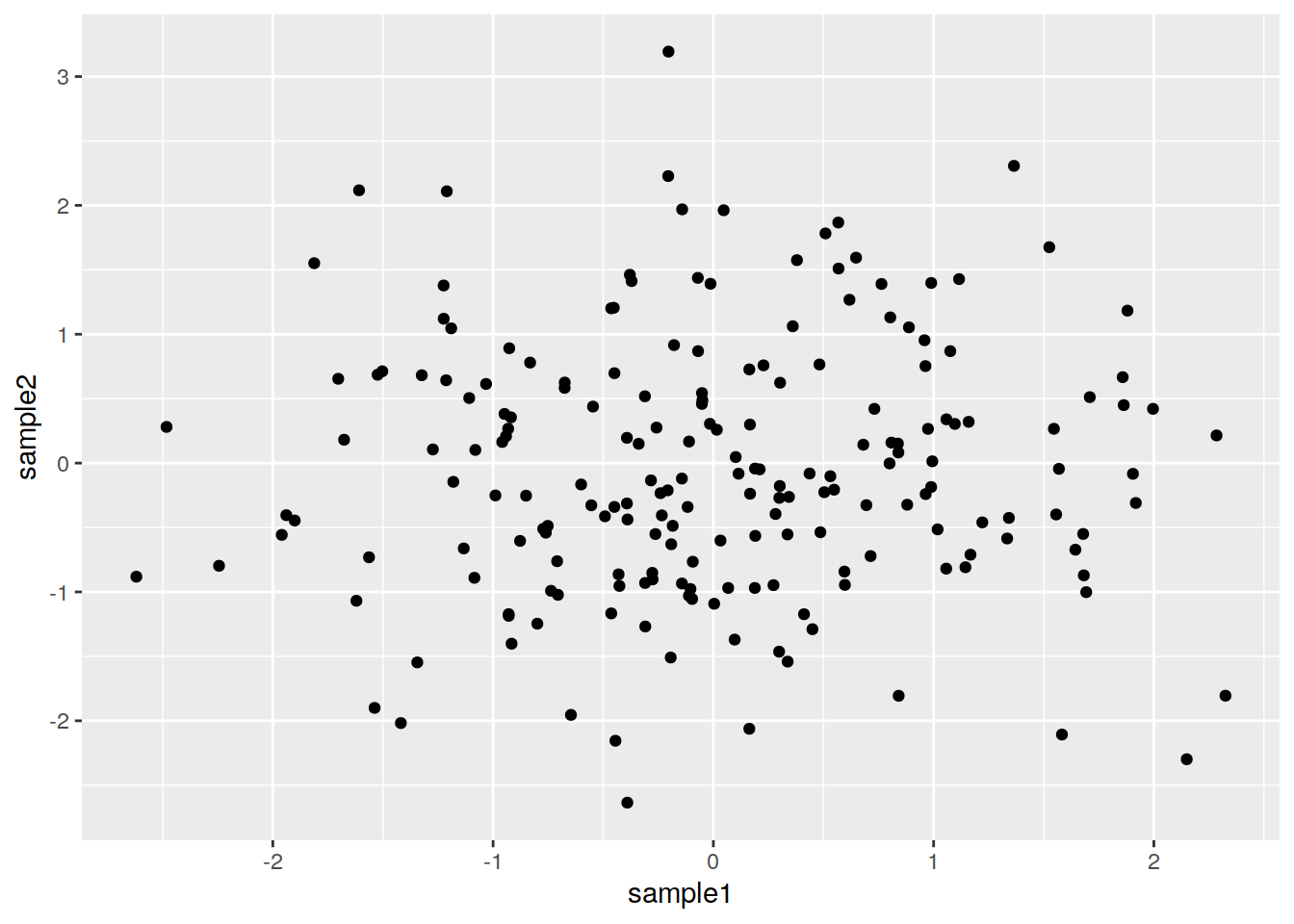• Add layers to that object to customize the plot:
• ggtitle to add a title
• geom_vline to add a vertical line
• etc.
``````ggplot(data= df1 , mapping=aes(x=sample1, y=sample2)) +
geom_point() +
ggtitle(label="my first ggplot") +
geom_vline(xintercept=0)``````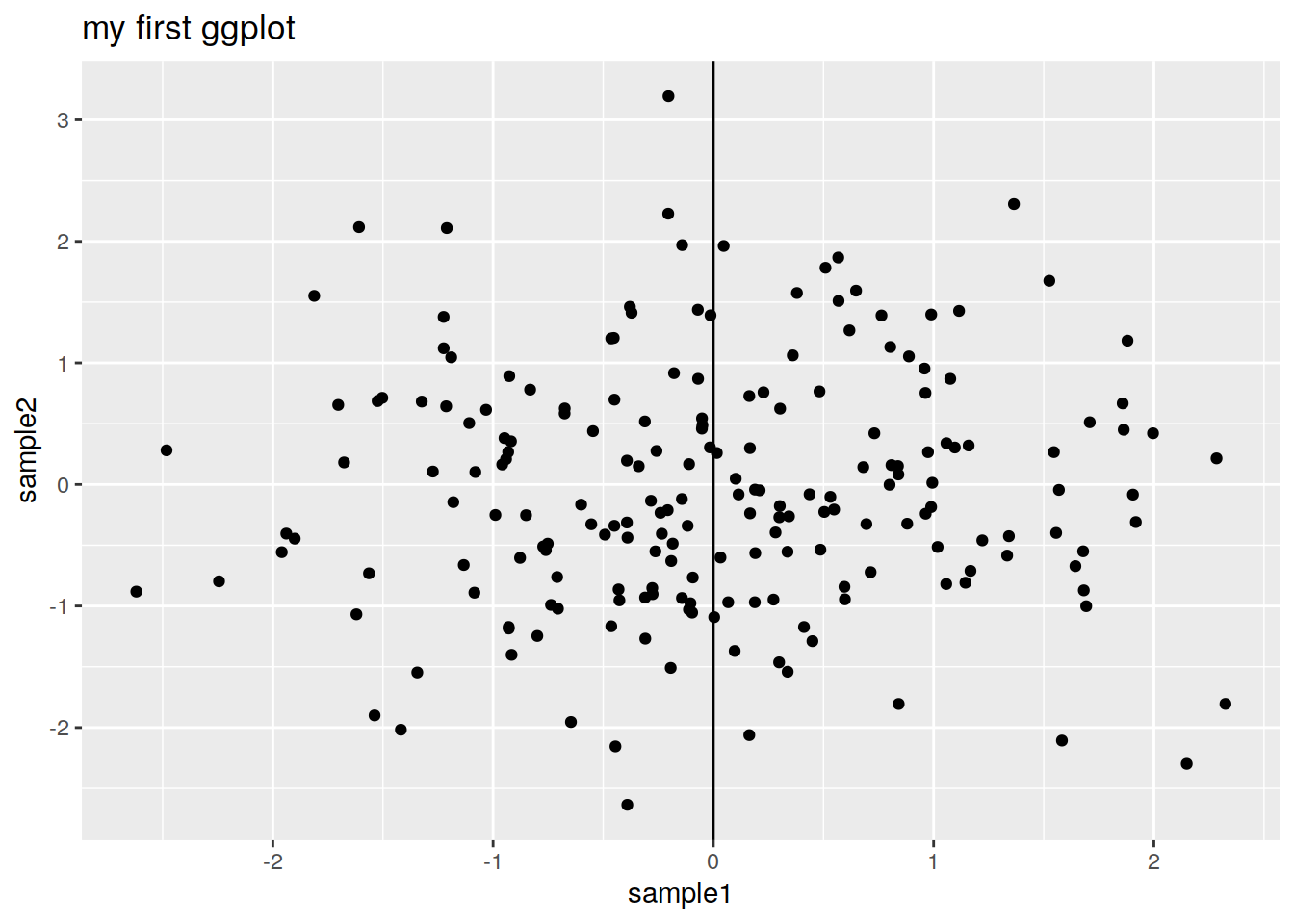Bookmark that ggplot2 reference and that good cheatsheet for some of the ggplot2 options.

• You can save the plot in an object at any time and add layers to that object:
``````# Save in an object
p <- ggplot(data= df1 , mapping=aes(x=sample1, y=sample2)) +
geom_point()
# Add layers to that object
p + ggtitle(label="my first ggplot")``````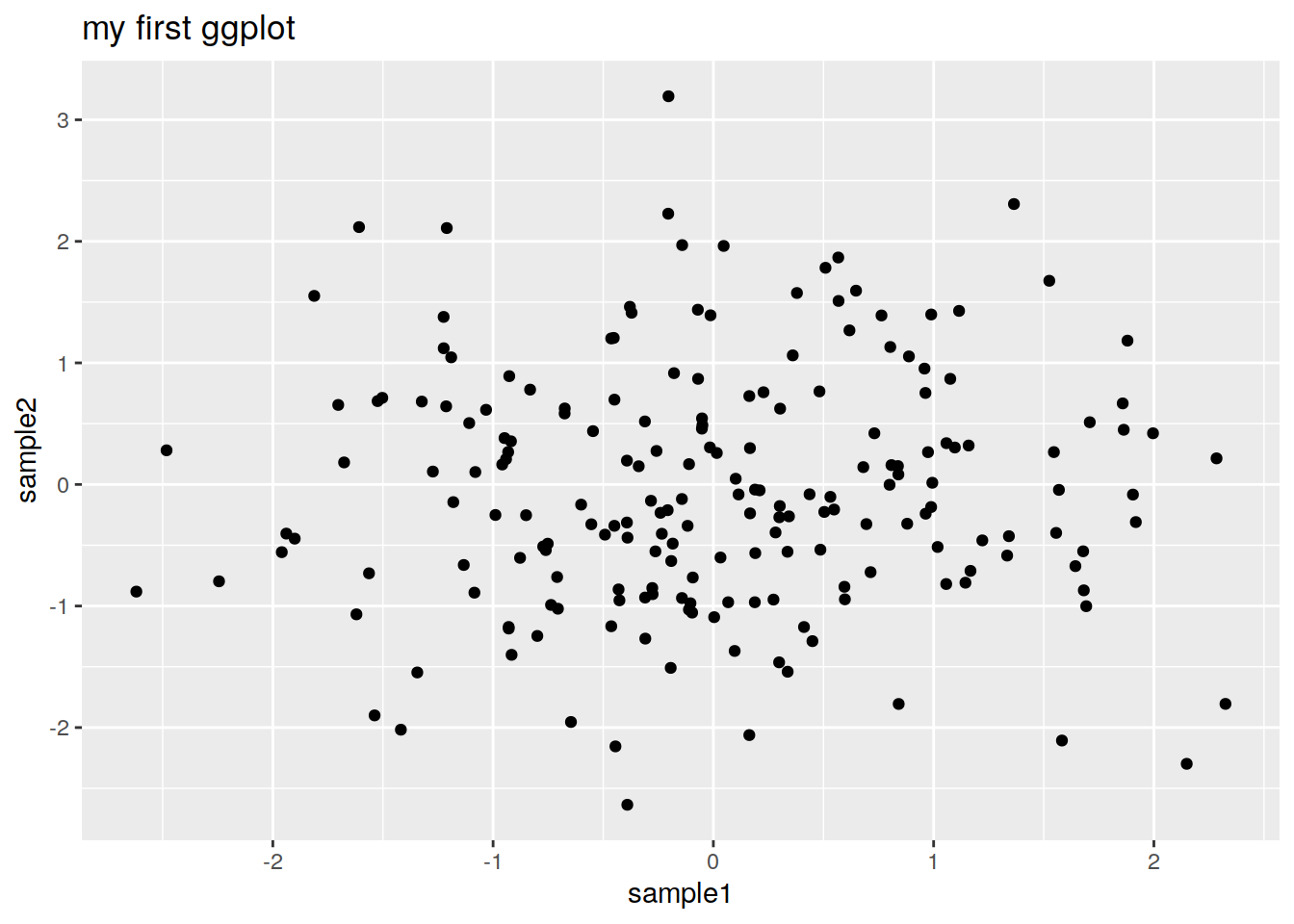• What is inside the aes (aesthetics)function ?
• Anything that varies according to your data !
• Columns with values to be plotted.
• Columns with which you want to, for example, color the points.

Color all points in red (not depending on the data):

``````ggplot(data=df1 , mapping=aes(x=sample1, y=sample2)) +
geom_point(color="red") ``````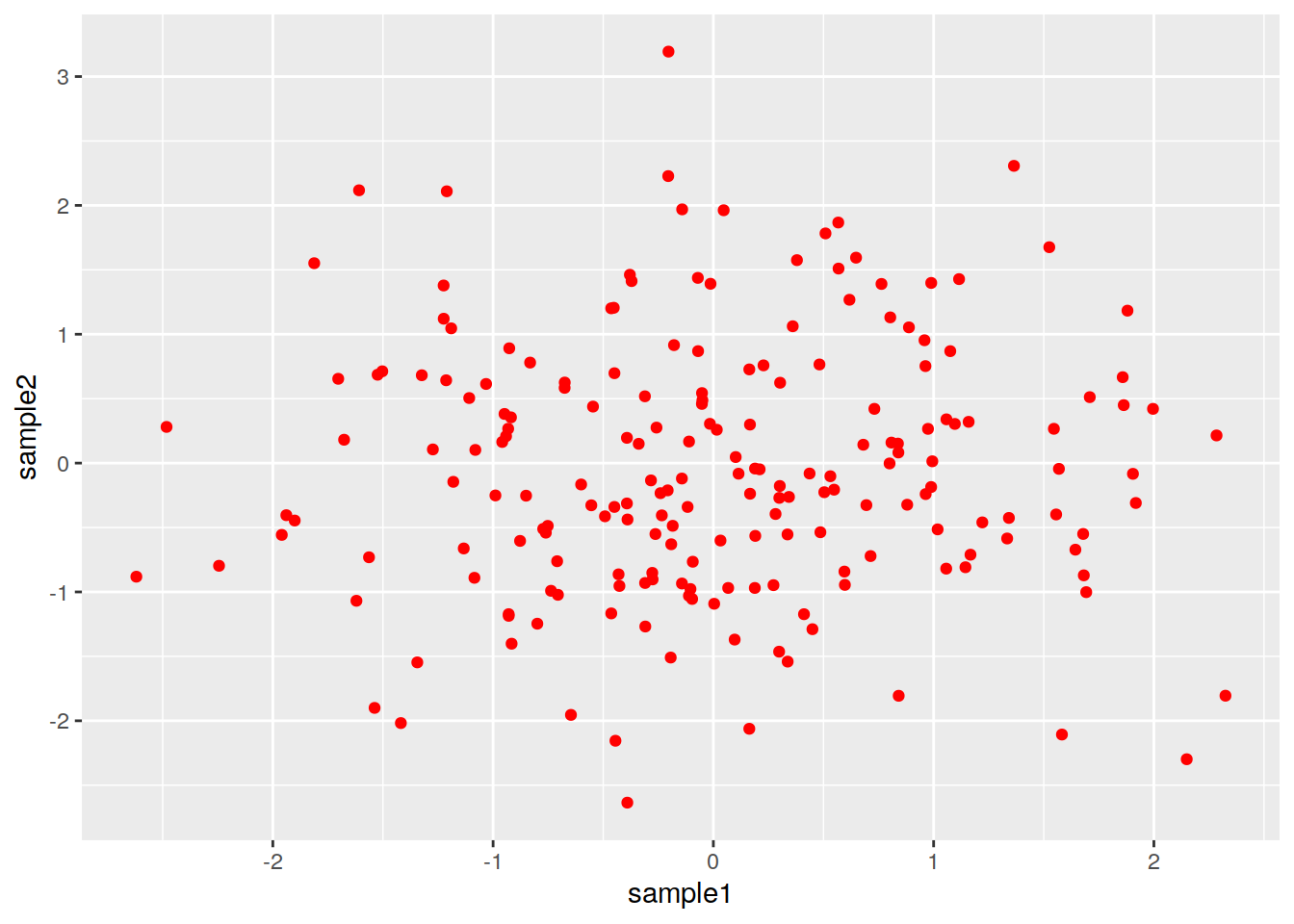Color the points according to another column in the data frame:

``````# Build a data frame from df1: add a column with "yes" and "no"
df2 <- data.frame(df1, grouping=rep(c("yes", "no"), c(80, 120)))

# Plot and add the color parameter in the aes():
pscat <- ggplot(data=df2, mapping=aes(x=sample1, y=sample2, color=grouping)) +
geom_point()
pscat``````